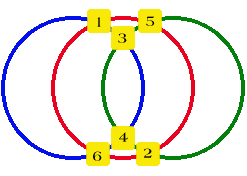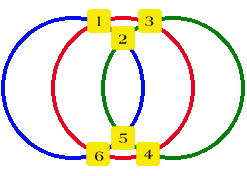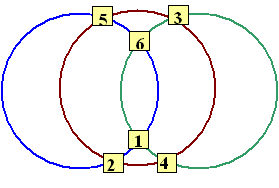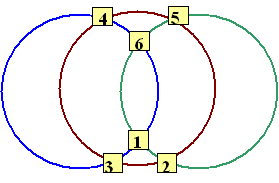#### You may also like### Prompt Cards

These two group activities use mathematical reasoning - one is numerical, one geometric.### Consecutive Numbers

An investigation involving adding and subtracting sets of consecutive numbers. Lots to find out, lots to explore.### Exploring Wild & Wonderful Number Patterns

EWWNP means Exploring Wild and Wonderful Number Patterns Created by Yourself! Investigate what happens if we create number patterns using some simple rules.

# Magic Circles

##### Age 7 to 11 Challenge Level:

Jason from Lark Rise Lower School has sent in a well explained answer.The rule for giving another set of numbers is to put odd numbers at the top in ascending order and the even numbers in descending order.

Josh from Ampthill, UK has quite a different way of doing it.In the top three boxes there is $1$, $2$, $3$. In the bottom three there is $6$, $5$, $4$, so each of the three pairs of numbers adds up to seven. (That is; $1$&$6$, $2$&$5$, $3$&$4$.)

Another solution from Sonya from Rocky Elementary School, Canada:And another from Year 6 at Brookfield School, UK: